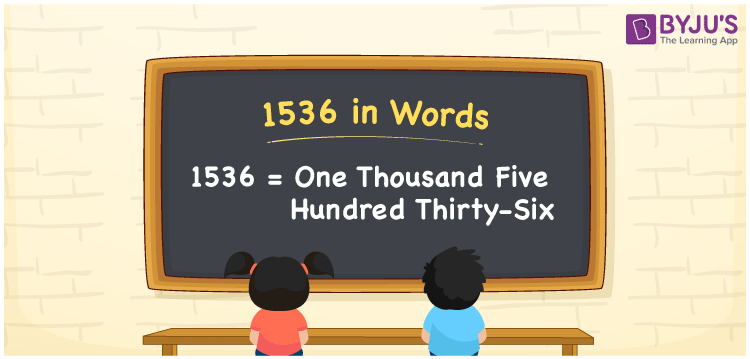# 1536 in Words

We can write 1536 in words as One Thousand Five Hundred Thirty-six. Suppose you have Rs. 1536 in your bank account, you can say that “I have One Thousand Five Hundred Thirty-six rupees in my bank account”. It is possible to convert 1536 into words with the help of a place value chart. Also, we know that 1536 is a cardinal number since it represents a specific quantity.

 1536 in words One Thousand Five Hundred Thirty-six One Thousand Five Hundred Thirty-six in Numbers 1536

## 1536 in English Words## How to Write 1536 in Words?

We can convert 1536 to words using a place value chart. This can be done as follows. The number 1536 has 4 digits, so let’s make a chart that shows the place value up to 4 digits.

 Thousands Hundreds Tens Ones 1 5 3 6

Thus, we can write the expanded form as:

1 × Thousand + 5 × Hundred + 3 × Ten + 6 × One

= 1 × 1000 + 5 × 100 + 3 × 10 + 6 × 1

= 1536

= One Thousand Five Hundred Thirty-six

1536 is the natural number that is succeeded by 1535 and preceded by 1537.

1536 in words – One Thousand Five Hundred Thirty-six

Is 1536 an odd number? – No.

Is 1536 an even number? – Yes

Is 1536 a perfect square number? – No

Is 1536 a perfect cube number? – No

Is 1536 a prime number? – No

Is 1536 a composite number? – Yes

## Frequently Asked Questions on 1536 in Words

Q1

### How to write 1536 in words?

1536 in English is written as “One Thousand Five Hundred Thirty-six”.
Q2

### Is 1536 a prime number?

No, the number 1536 is not a prime number. One Thousand Five Hundred Thirty-six is a composite number. Because 1536 has more divisors than 1 and itself.
Q3

### Write One Thousand Five Hundred Thirty-six in numbers.

One Thousand Five Hundred Thirty-six in numbers is 1536.